基于S函数的BP神经网络PID控制器及simulink仿真
文章来源和摘要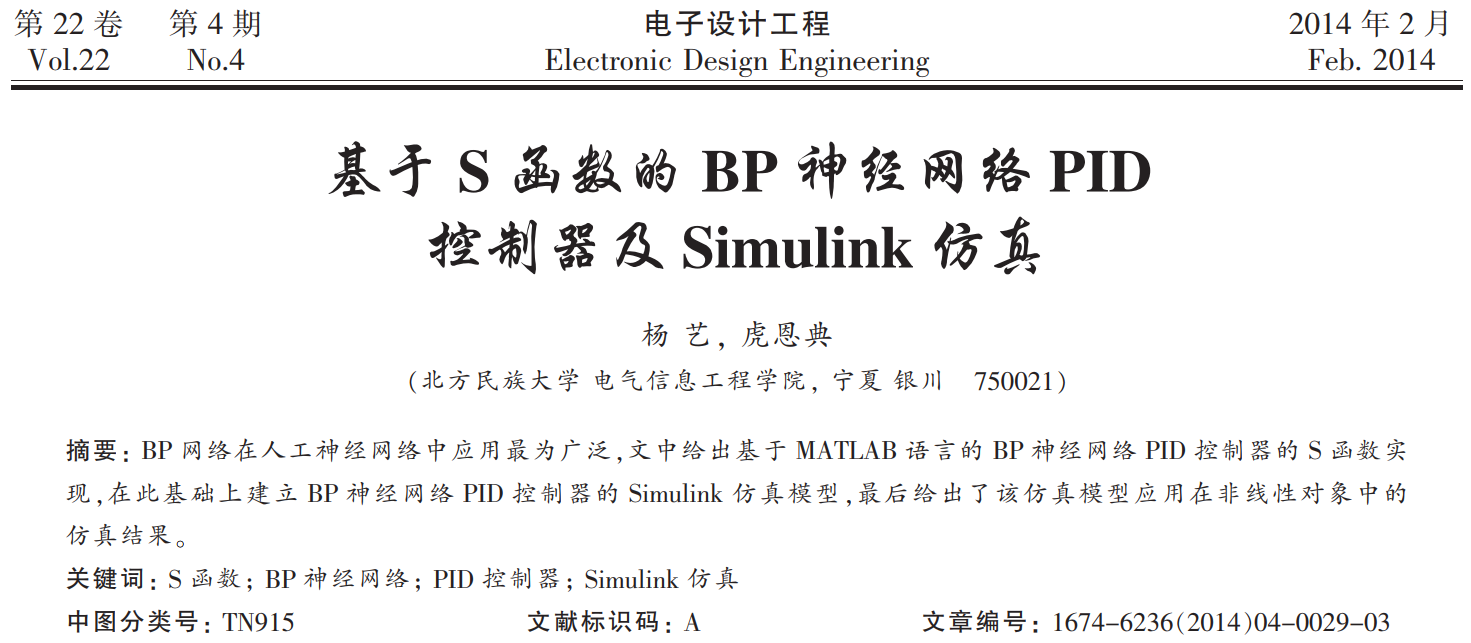S函数的编写格式和运行步骤
S函数的编写格式为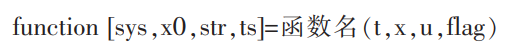各个变量对应的含义分别为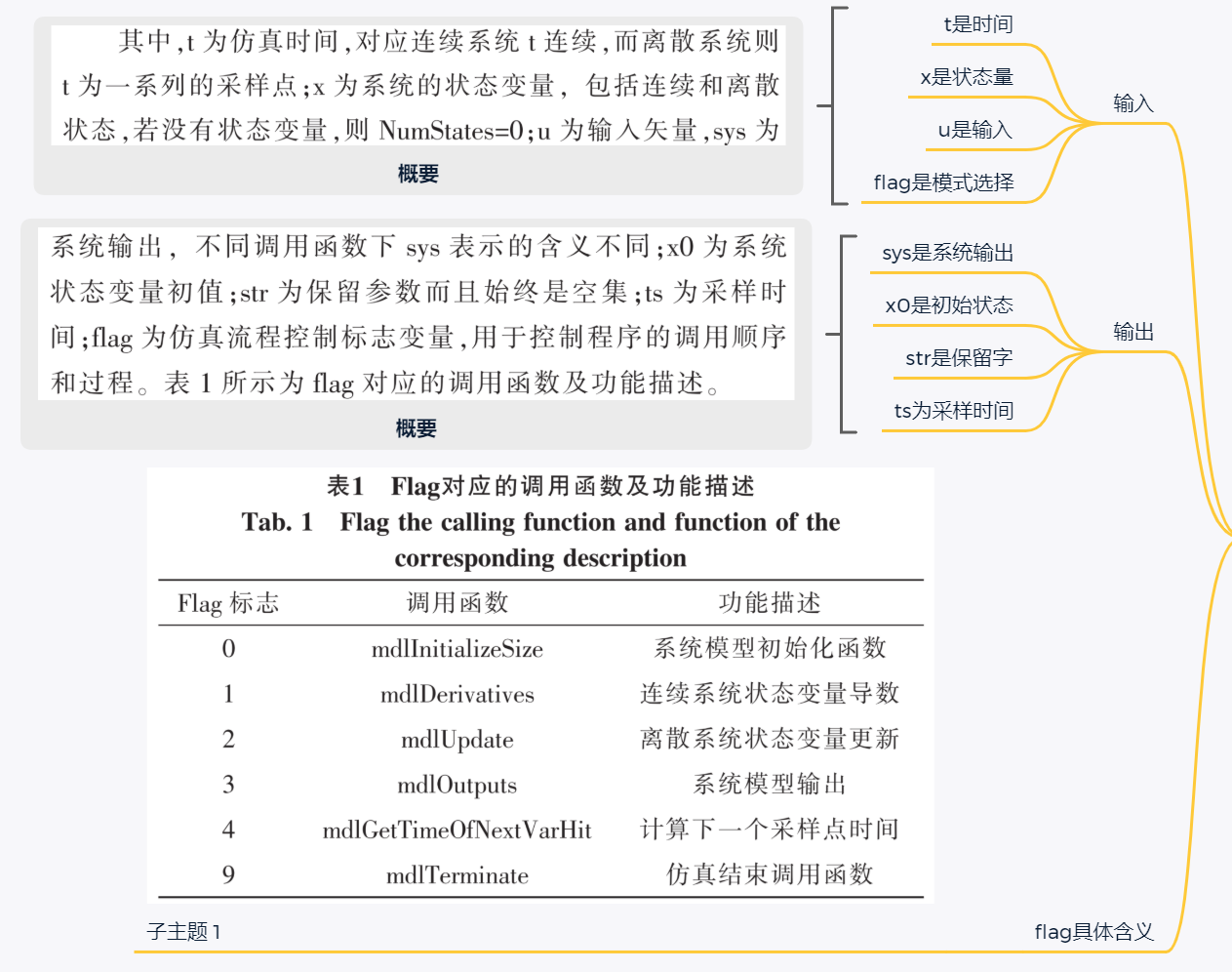运行步骤：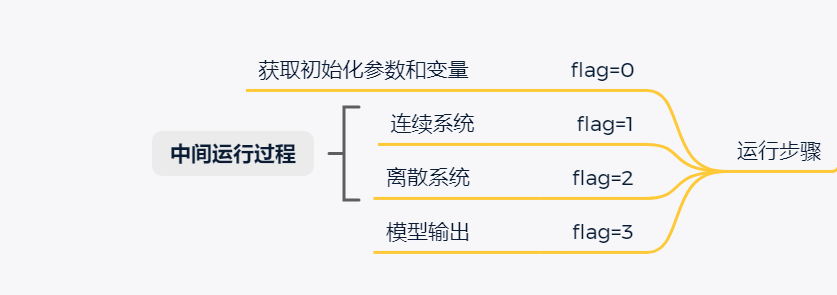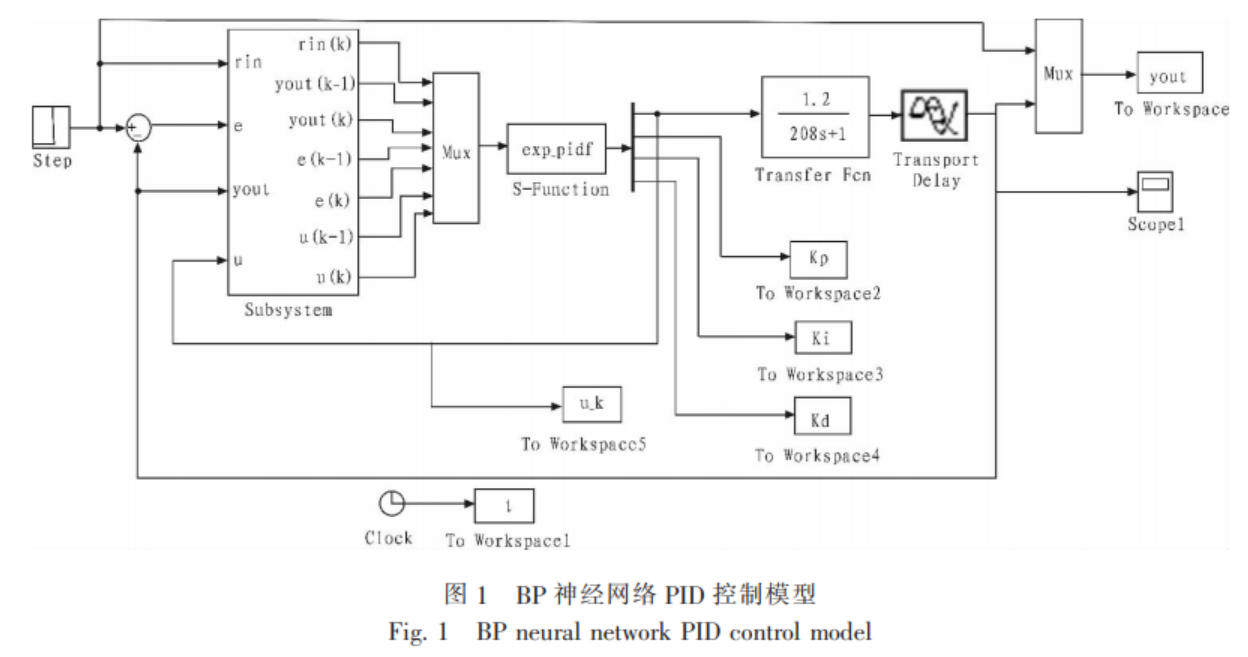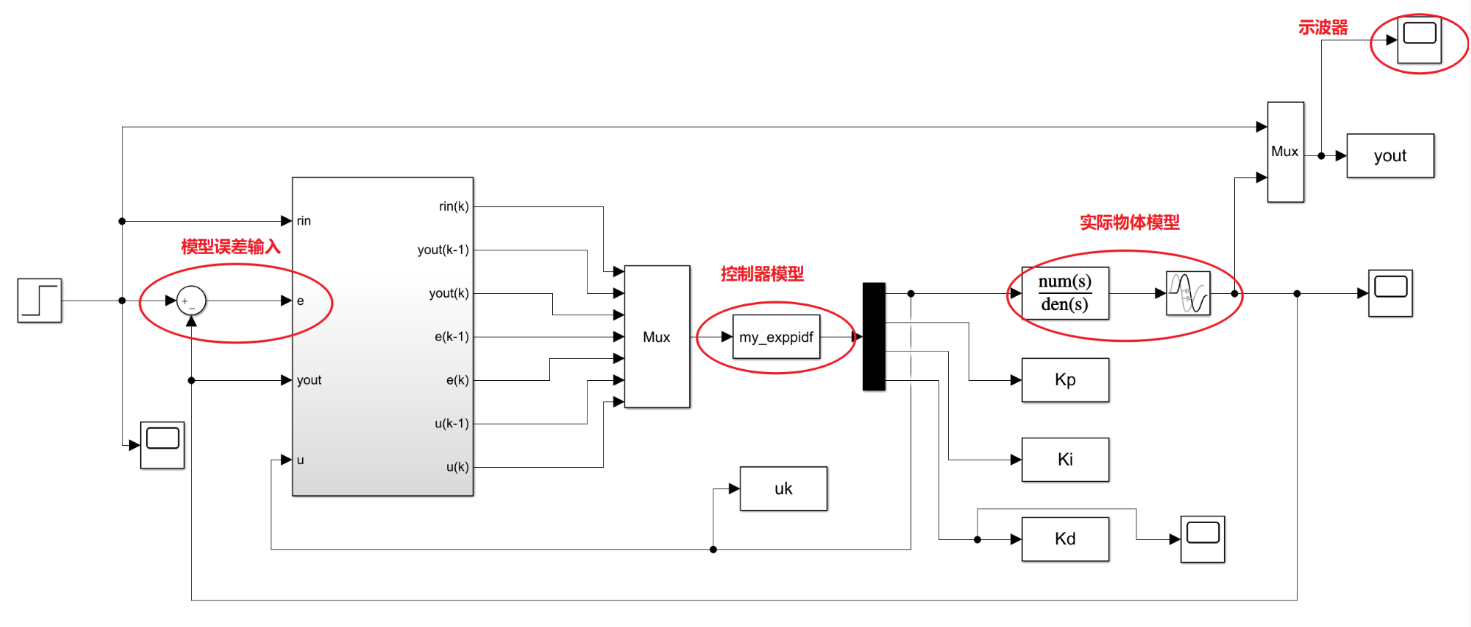其中mux的作用是把常量合并成数组
demux（黑色部分）的作用是吧一个数组重新解析成标量
在这里有一个疑惑，就是输入的子系统中，并没有设置有效的单位延时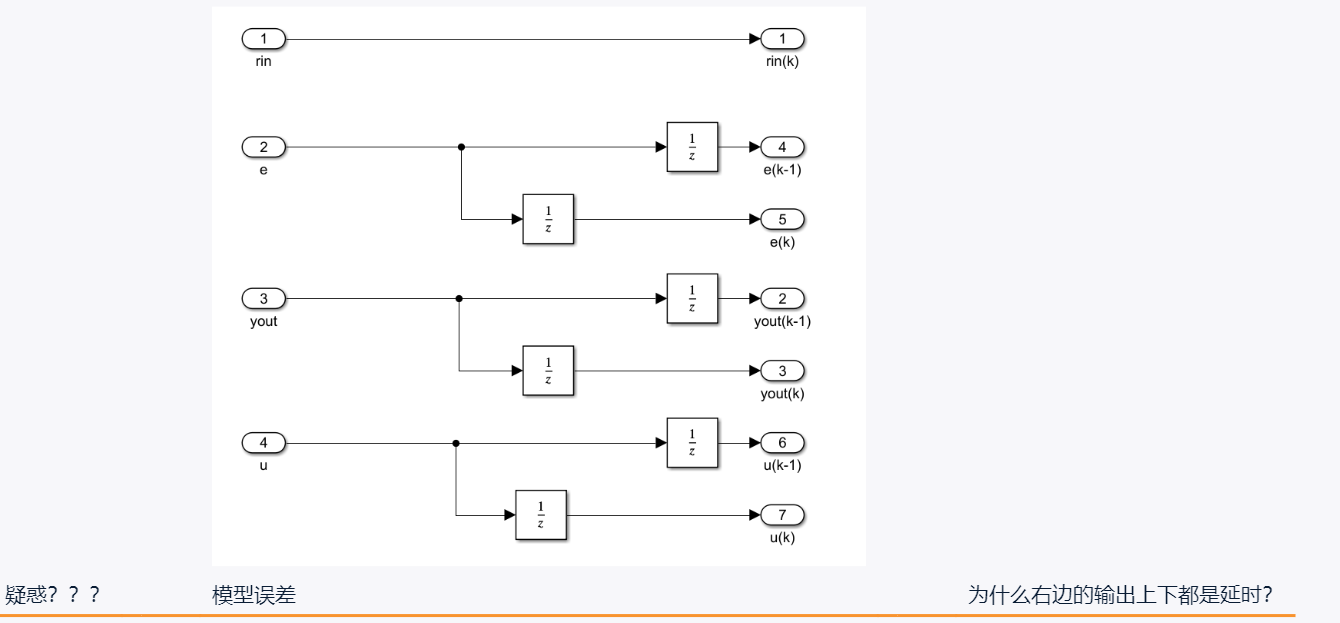这篇博客的评论区里，大家普遍认为有造假的嫌疑，我也十分困惑。控制器部分，利用S函数实现BP神经网络的PID。论文给出的仿真效果如下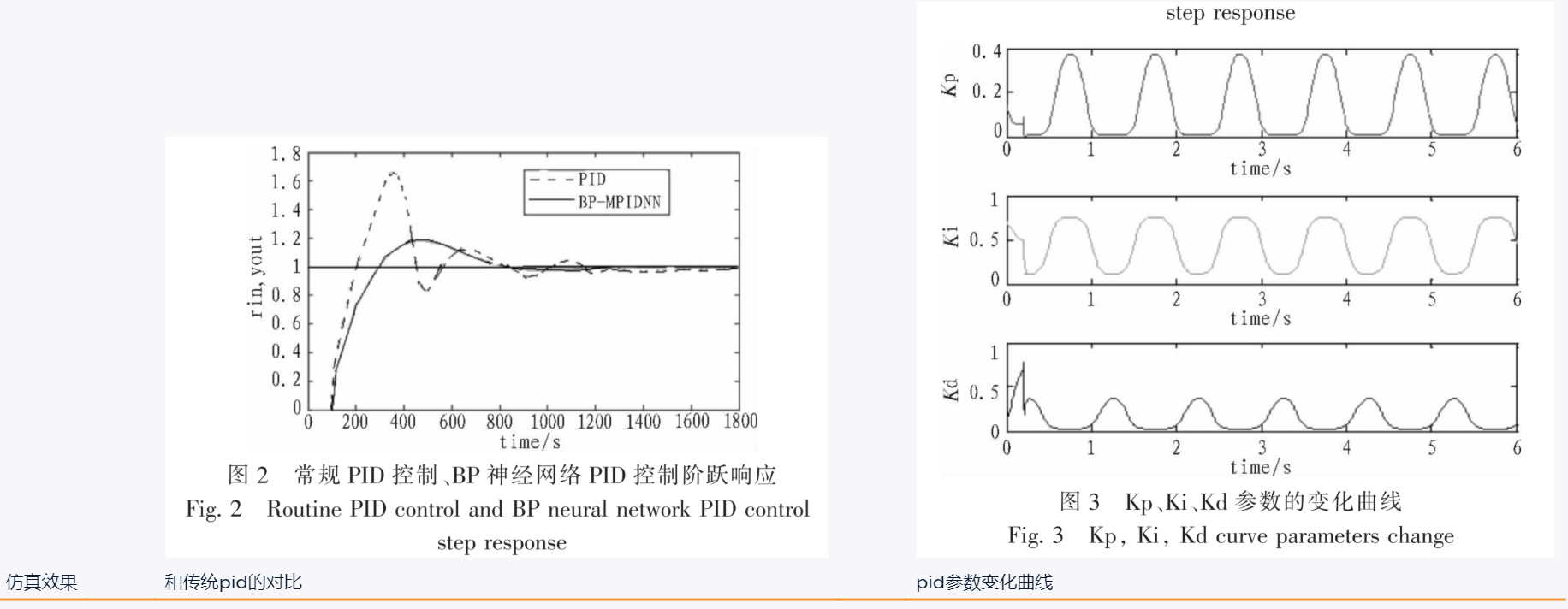实际的控制器仿真效果如图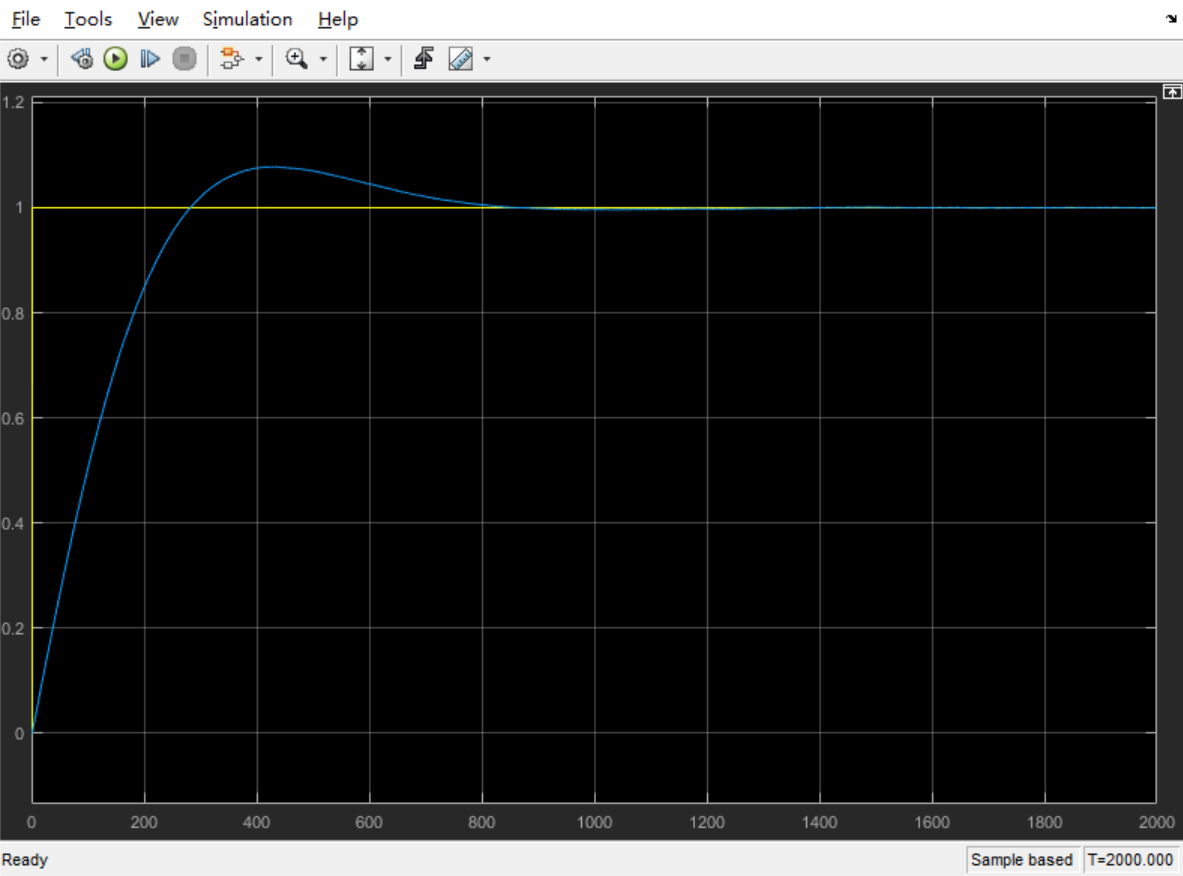PID的输出参数变化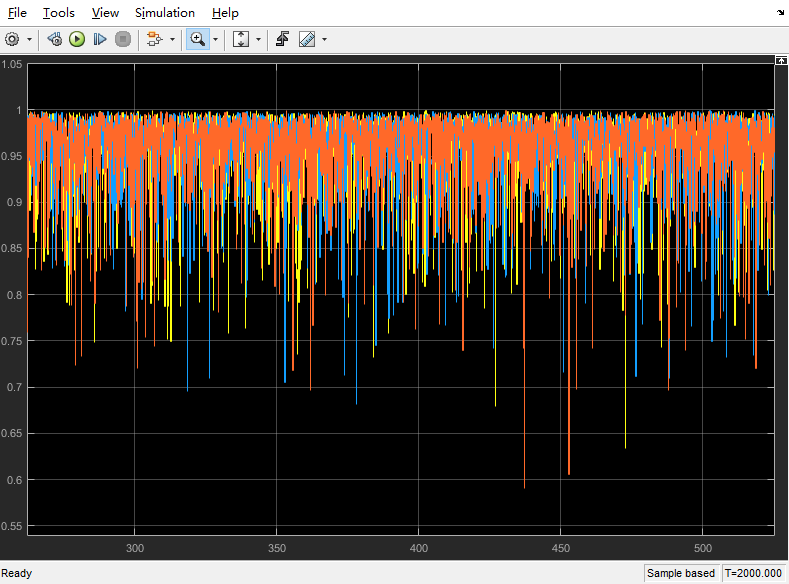S函数模型初始化部分代码理解
S函数总共分为4个部分，第一部分为切换函数，第二部分为初始化，第三部分为数据更新部分，第四部分为模型输出。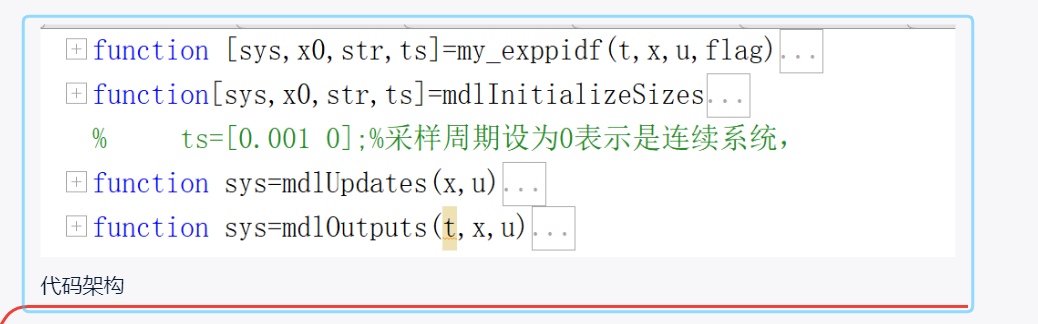% 模型初始化
function[sys,x0,str,ts]=mdlInitializeSizes
sizes=simsizes;%用于设置模块参数的结构体用simsizes来生成
sizes.NumContStates=0;%模块连续状态变量的个数
sizes.NumDiscStates=3;%模块离散状态变量的个数
sizes.NumOutputs=4;%模块输出变量的个数
sizes.NumInputs=7;%模块输入变量的个数
sizes.DirFeedthrough=1;%模块是否存在直接贯通，1表示存在直接贯通，若为0，则mdlOutputs函数里不能有u
sizes.NumSampleTimes=1;%模块的采样时间个数,至少是一个
sys=simsizes(sizes);%设置完后赋给sys输出
x0=zeros(3,1);%系统状态变量设置，3行一列
str=[];
ts=[0 0];%采样周期设为0表示是连续系统，
%     ts=[0.001 0];%采样周期设为0表示是连续系统，

simsizes是什么，帮助文档给出如下

ans =
包含以下字段的 struct:
NumContStates: 0
NumDiscStates: 0
NumOutputs: 0
NumInputs: 0
DirFeedthrough: 0
NumSampleTimes: 0


说明是一个系统的默认变量，不需要初始化就能直接引用。
S函数模型更新部分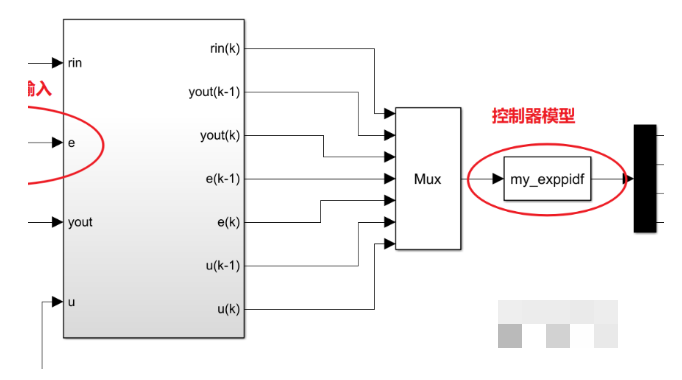% 模型更新部分，输入为系统状态量和系统输入
T=0.001;
x=[u(5);x(2)+u(5)*T;(u(5)-u(4))/T];%3个状态量（偏差、偏差和以及偏差变化量），u(5)是偏差，u(4)是上一次的偏差，x(2)则是之前的偏差和
sys=[x(1);x(2);x(3)];

x和u分别是什么？
u应该就是mux左侧的那7个输入变量，实际对应u(1)~u(7)
在这之前，初始化的时候，x0=zero(3,1)=[0;0;0]，这里的x正式成为状态量，分别为偏差，偏差和，偏差变化量。
所以模型更新部分实际只更新了状态量。
S函数模型输出部分
参数的初始化
% 这一段基本上都是一些参数的初始化
xite=0.2;   % 学习速率
alfa=0.05;  % 惯性因子
IN=3;H=5;OUT=3; % 神经网络的3-5-3架构

% 输入层-隐含层的加权系数
wi=rand(5,3);%产生一个5*3的随机数矩阵，随机数在（0，1）区间
wi_1=wi;wi_2=wi;wi_3=wi;    % 隐含层加权系数
% 隐含层-输出层的加权系数
wo=rand(3,5);
wo_1=wo;wo_2=wo;wo_3=wo;    % 输出层加权系数
Oh=zeros(5,1);  %产生一个1*5的零矩阵(行矩阵)，这里就是隐含层
I=Oh;
% 输入层的具体输入
xi=[u(1),u(3),u(5)];%神经网络训练的3个输入，期望值、误差以及实际值
% PID的3个对应参量
epid=[x(1);x(2);x(3)];%3个状态变量（偏差、偏差和、偏差变化量）（3*1矩阵，列向量）
I=xi*wi';%隐层的输入

接着进行隐含层节点和输出层节点值的更新
% 更新隐含层
for j=1:1:5
% 隐含层的节点值
Oh(j)=(exp(I(j))-exp(-I(j)))/(exp(I(j))+exp(-I(j)));%隐层的输出值（1*5矩阵）行矩阵
end
% 更新输出层
K1=wo*Oh;   % 输出层的输入值（3*1矩阵）
for i=1:1:3
% 输出层的输出值
K(i)=exp(K1(i))/(exp(K1(i))+exp(-K1(i)));%得到输出层的输出（KP、KI、KD）（1*3矩阵，行向量）
end
% 这里得到的K相当于是pid的3个参数

% 最终控制器的完全输出值
u_k=K*epid;%计算得到控制律u，1个值

最后，根据反向传播算法进行权值的调整
 %隐含层至输出层的权值调整
dyu=sign((u(3)-u(2))/(u(7)-u(6)+0.0001));
for j=1:1:3
dK(j)=2/(exp(K1(j))+exp(-K1(j)))^2; %输出层的输出的一阶导
end
for i=1:1:3
delta3(i)=u(5)*dyu*epid(i)*dK(i);  %输出层的delta
end
for j=1:1:3
for i=1:1:5
d_wo=xite*delta3(j)*Oh(i)+alfa*(wo_1-wo_2);
end
end
% 隐含层-输出层的加权系数的调整
wo=wo_1+d_wo;

%以下是输入层至隐含层的权值调整
for i=1:1:5
dO(i)=4/(exp(I(i))+exp(-I(i)))^2;%(1*5矩阵)
end
segma=delta3*wo;%（1*5矩阵，行向量）
delta2 = dO.*segma;
d_wi = delta2'*xi+alfa*(wi_1-wi_2);
% 输入层-隐含层的加权系数调整
wi=wi_1+d_wi;

% 这里猜测存储的是历史信息？
wo_3=wo_2;
wo_2=wo_1;
wo_1=wo;%储存输出层本次调整后的权值

wi_3=wi_2;
wi_2=wi_1;
wi_1=wi;%储存隐层本次调整后的权值

Kp=K(1);Ki=K(2);Kd=K(3);
sys=[u_k,Kp,Ki,Kd];

S函数完整代码附录
% 有个疑惑，到底是什么在选择flag？为什么这里没有主函数？
function [sys,x0,str,ts]=my_exppidf(t,x,u,flag)
switch flag,
case 0,
[sys,x0,str,ts]=mdlInitializeSizes;
case 2,
case 3,
sys=mdlOutputs(t,x,u);
case {1,4,9},
sys=[];
otherwise
error(['unhandled flag=',num2str(flag)]);%异常处理
end

% 模型初始化
function[sys,x0,str,ts]=mdlInitializeSizes
sizes=simsizes;%用于设置模块参数的结构体用simsizes来生成
sizes.NumContStates=0;%模块连续状态变量的个数
sizes.NumDiscStates=3;%模块离散状态变量的个数
sizes.NumOutputs=4;%模块输出变量的个数
sizes.NumInputs=7;%模块输入变量的个数
sizes.DirFeedthrough=1;%模块是否存在直接贯通，1表示存在直接贯通，若为0，则mdlOutputs函数里不能有u
sizes.NumSampleTimes=1;%模块的采样时间个数,至少是一个
sys=simsizes(sizes);%设置完后赋给sys输出
x0=zeros(3,1);%系统状态变量设置
str=[];
ts=[0 0];%采样周期设为0表示是连续系统，
%     ts=[0.001 0];%采样周期设为0表示是连续系统，
T=0.001;
x=[u(5);x(2)+u(5)*T;(u(5)-u(4))/T];%3个状态量（偏差、偏差和以及偏差变化量），u(5)是偏差，u(4)是上一次的偏差，x(2)则是之前的偏差和
sys=[x(1);x(2);x(3)];
function sys=mdlOutputs(t,x,u)
xite=0.2;
alfa=0.05;
IN=3;H=5;OUT=3;
wi=rand(5,3);%产生一个5*3的随机数矩阵，随机数在（0，1）区间
wi_1=wi;wi_2=wi;wi_3=wi;
wo=rand(3,5);
wo_1=wo;wo_2=wo;wo_3=wo;
Oh=zeros(5,1);%产生一个1*5的零矩阵(行矩阵)
I=Oh;
xi=[u(1),u(3),u(5)];%神经网络训练的3个输入，期望值、误差以及实际值
epid=[x(1);x(2);x(3)];%3个状态变量（偏差、偏差和、偏差变化量）（3*1矩阵，列向量）
I=xi*wi';%隐层的输入
for j=1:1:5
Oh(j)=(exp(I(j))-exp(-I(j)))/(exp(I(j))+exp(-I(j)));%隐层的输出值（1*5矩阵）行矩阵
end
K1=wo*Oh;%输出层的输入（3*1矩阵）
for i=1:1:3
K(i)=exp(K1(i))/(exp(K1(i))+exp(-K1(i)));%得到输出层的输出（KP、KI、KD）（1*3矩阵，行向量）
end
u_k=K*epid;%计算得到控制律u，1个值
%%以下是权值调整
%隐含层至输出层的权值调整
dyu=sign((u(3)-u(2))/(u(7)-u(6)+0.0001));
for j=1:1:3
dK(j)=2/(exp(K1(j))+exp(-K1(j)))^2; %输出层的输出的一阶导
end
for i=1:1:3
delta3(i)=u(5)*dyu*epid(i)*dK(i);  %输出层的delta
end
for j=1:1:3
for i=1:1:5
d_wo=xite*delta3(j)*Oh(i)+alfa*(wo_1-wo_2);
end
end
wo=wo_1+d_wo;
%以下是输入层至隐含层的权值调整
for i=1:1:5
dO(i)=4/(exp(I(i))+exp(-I(i)))^2;%(1*5矩阵)
end
segma=delta3*wo;%（1*5矩阵，行向量）
delta2 = dO.*segma;
d_wi = delta2'*xi+alfa*(wi_1-wi_2);
wi=wi_1+d_wi;
wo_3=wo_2;
wo_2=wo_1;
wo_1=wo;%储存输出层本次调整后的权值
wi_3=wi_2;
wi_2=wi_1;
wi_1=wi;%储存隐层本次调整后的权值
Kp=K(1);Ki=K(2);Kd=K(3);
sys=[u_k,Kp,Ki,Kd];

压缩包截图展开全文万次阅读 多人点赞 2018-06-05 20:59:48
      最近看到杨艺的那篇《基于S函数的BP神经网络PID控制器及simulink仿真》，也想是否能够实现Simulink仿真，于是上网搜索相关资料，发现原来有许多前辈（1点击打开链接 2 点击打开链接 3点击打开链接）早已经做过类似的工作了，并且总结出许多经验和错误，但鉴于在论坛中许多错误没有一个完整的回答，信息都是零零散散，于是我打算把我做的一整套流程记录下来，以免日后忘记，同时也希望可以帮助到一些人。      对于链接1里面是我最早看到的资料，前辈1在MATLAB中文论坛中发布自己搭建的工程文件及其所遇到的问题，后面楼主说是解决了问题，但是没有上传（这个可以谅解），另外里面有许多热心网友的回答是非常有用的（这些回答对我自己后面解决相关问题，成功实现Simulink仿真是非常关键的，在这里非常感谢论坛中的各位前辈）。     对于链接2这个确实是可以用的，只不过对MATLAB版本有限制。开始我下载之后用我的MATLAB2016b打开工程，点击run后就报错，The block 'BPPID/Subsystem/Unit Delay1' does not permit continuous sample time (0 or [0,0]) for the parameter 'SampleTime'.里面分了两大派，一部分人说亲测有效，大赞跟帖；一部分人也像我一样运行报错，此时我疑惑了。于是猜测可能是MATLAB版本问题，于是在另一台电脑上的MATLAB2014b版本运行，果然得到楼主那一条漂亮的曲线（虽然我的工作就是点击一下run，但是还是很开心）。但鉴于我的电脑安装的是MATLAB2016b版本，不可能让我卸载重装2014版吧。于是琢磨怎么将其移植到MATLAB2016b版本上。      对于链接3非常感谢这个楼主的S函数代码，里面注释很详细，我也是在这个楼主的基础上修改的（非常感谢）。接下来我将把我调试的整个流程做如下介绍：1、编写S函数（感谢上面三位楼主，如有侵权，通知我撤销）function [sys,x0,str,ts]=my_exppidf(t,x,u,flag)
switch flag,
case 0,
[sys,x0,str,ts]=mdlInitializeSizes;
case 2,
case 3,
sys=mdlOutputs(t,x,u);
case {1,4,9},
sys=[];
otherwise
error(['unhandled flag=',num2str(flag)]);%异常处理
end
function[sys,x0,str,ts]=mdlInitializeSizes
sizes=simsizes;%用于设置模块参数的结构体用simsizes来生成
sizes.NumContStates=0;%模块连续状态变量的个数
sizes.NumDiscStates=3;%模块离散状态变量的个数
sizes.NumOutputs=4;%模块输出变量的个数
sizes.NumInputs=7;%模块输入变量的个数
sizes.DirFeedthrough=1;%模块是否存在直接贯通，1表示存在直接贯通，若为0，则mdlOutputs函数里不能有u
sizes.NumSampleTimes=1;%模块的采样时间个数,至少是一个
sys=simsizes(sizes);%设置完后赋给sys输出
x0=zeros(3,1);%系统状态变量设置
str=[];
ts=[0 0];%采样周期设为0表示是连续系统，
%     ts=[0.001 0];%采样周期设为0表示是连续系统，
T=0.001;
x=[u(5);x(2)+u(5)*T;(u(5)-u(4))/T];%3个状态量（偏差、偏差和以及偏差变化量），u(5)是偏差，u(4)是上一次的偏差，x(2)则是之前的偏差和
sys=[x(1);x(2);x(3)];
function sys=mdlOutputs(t,x,u)
xite=0.2;
alfa=0.05;
IN=3;H=5;OUT=3;
wi=rand(5,3);%产生一个5*3的随机数矩阵，随机数在（0，1）区间
wi_1=wi;wi_2=wi;wi_3=wi;
wo=rand(3,5);
wo_1=wo;wo_2=wo;wo_3=wo;
Oh=zeros(5,1);%产生一个1*5的零矩阵(行矩阵)
I=Oh;
xi=[u(1),u(3),u(5)];%神经网络训练的3个输入，期望值、误差以及实际值
epid=[x(1);x(2);x(3)];%3个状态变量（偏差、偏差和、偏差变化量）（3*1矩阵，列向量）
I=xi*wi';%隐层的输入
for j=1:1:5
Oh(j)=(exp(I(j))-exp(-I(j)))/(exp(I(j))+exp(-I(j)));%隐层的输出值（1*5矩阵）行矩阵
end
K1=wo*Oh;%输出层的输入（3*1矩阵）
for i=1:1:3
K(i)=exp(K1(i))/(exp(K1(i))+exp(-K1(i)));%得到输出层的输出（KP、KI、KD）（1*3矩阵，行向量）
end
u_k=K*epid;%计算得到控制律u，1个值
%%以下是权值调整
%隐含层至输出层的权值调整
dyu=sign((u(3)-u(2))/(u(7)-u(6)+0.0001));
for j=1:1:3
dK(j)=2/(exp(K1(j))+exp(-K1(j)))^2; %输出层的输出的一阶导
end
for i=1:1:3
delta3(i)=u(5)*dyu*epid(i)*dK(i);  %输出层的delta
end
for j=1:1:3
for i=1:1:5
d_wo=xite*delta3(j)*Oh(i)+alfa*(wo_1-wo_2);
end
end
wo=wo_1+d_wo;
%以下是输入层至隐含层的权值调整
for i=1:1:5
dO(i)=4/(exp(I(i))+exp(-I(i)))^2;%(1*5矩阵)
end
segma=delta3*wo;%（1*5矩阵，行向量）
delta2 = dO.*segma;
d_wi = delta2'*xi+alfa*(wi_1-wi_2);
wi=wi_1+d_wi;
wo_3=wo_2;
wo_2=wo_1;
wo_1=wo;%储存输出层本次调整后的权值
wi_3=wi_2;
wi_2=wi_1;
wi_1=wi;%储存隐层本次调整后的权值
Kp=K(1);Ki=K(2);Kd=K(3);
sys=[u_k,Kp,Ki,Kd];       2、搭建Simulink模型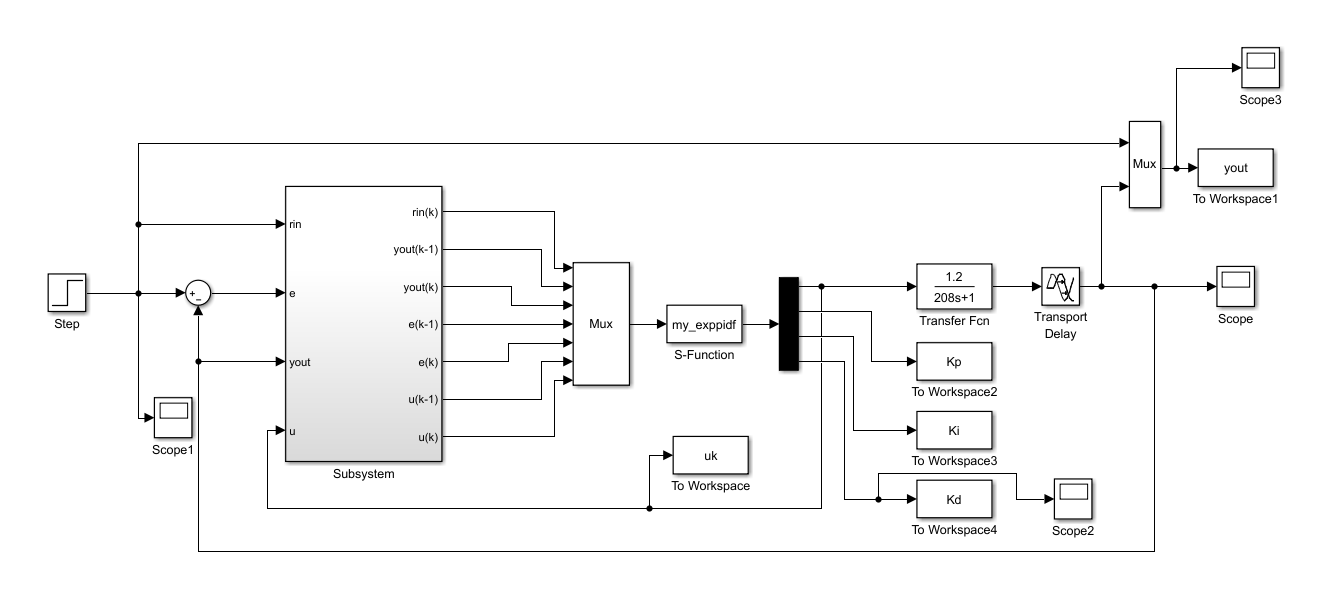其中Subsystem为：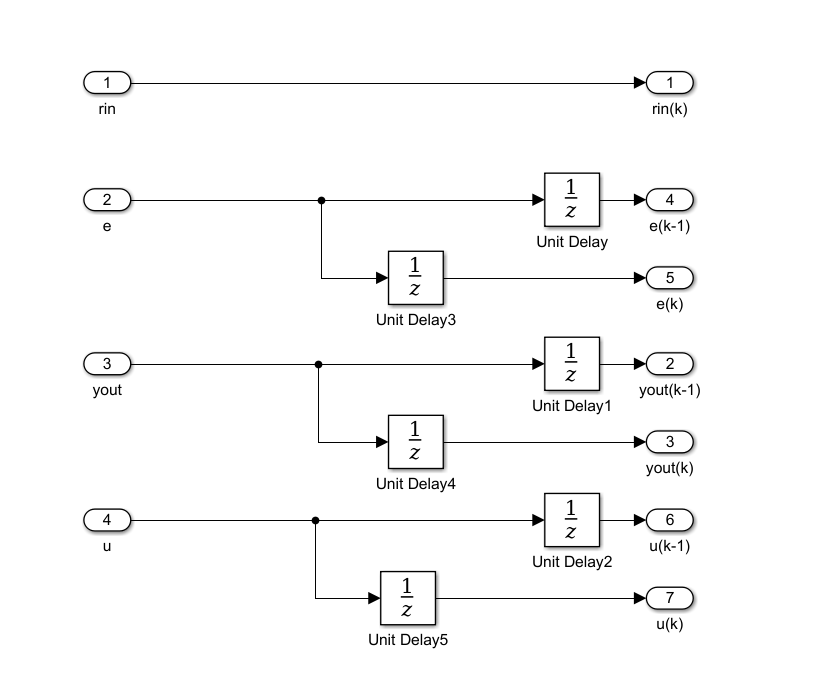3、相关参数配置     3.1 系统参数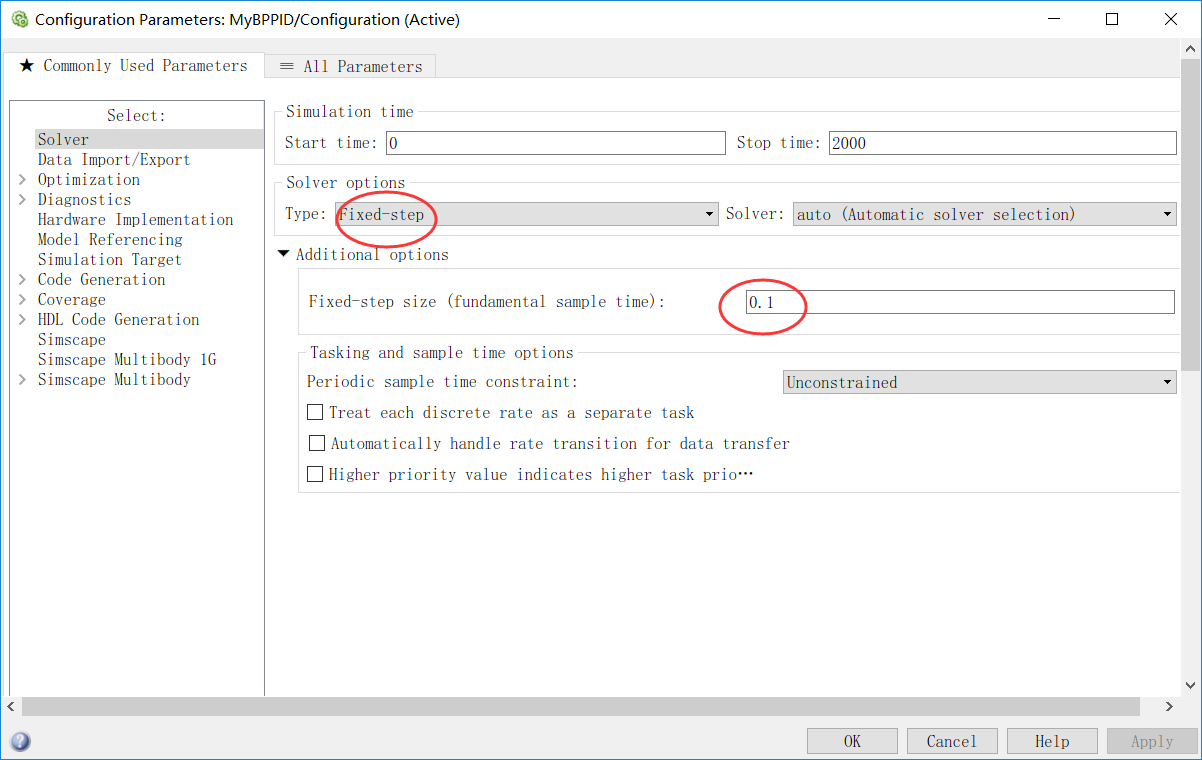3.2  Transport Delay 参数设置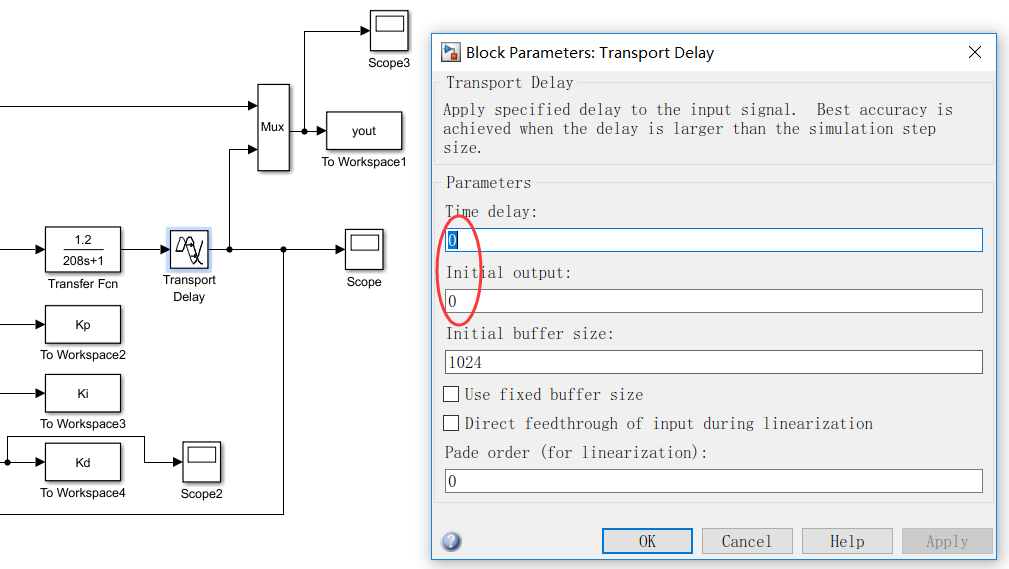3.3  Unit Delay 参数设置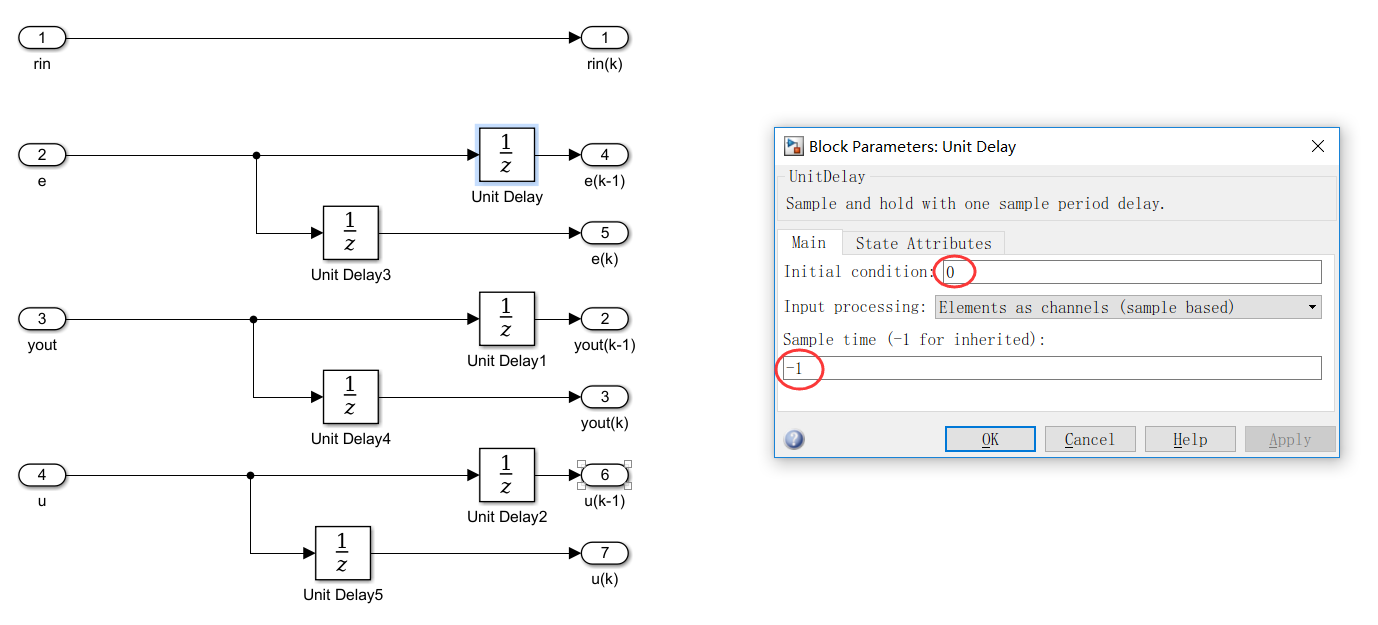tips：其中 系统参数fundamental sample time设置 与 Transport Delay 参数 Time delay 有联系，如果设置不好则会出现输出一直为0的情况。4、实验结果     4.1 当fundamental sample time 设置为0.02时，实验结果如下：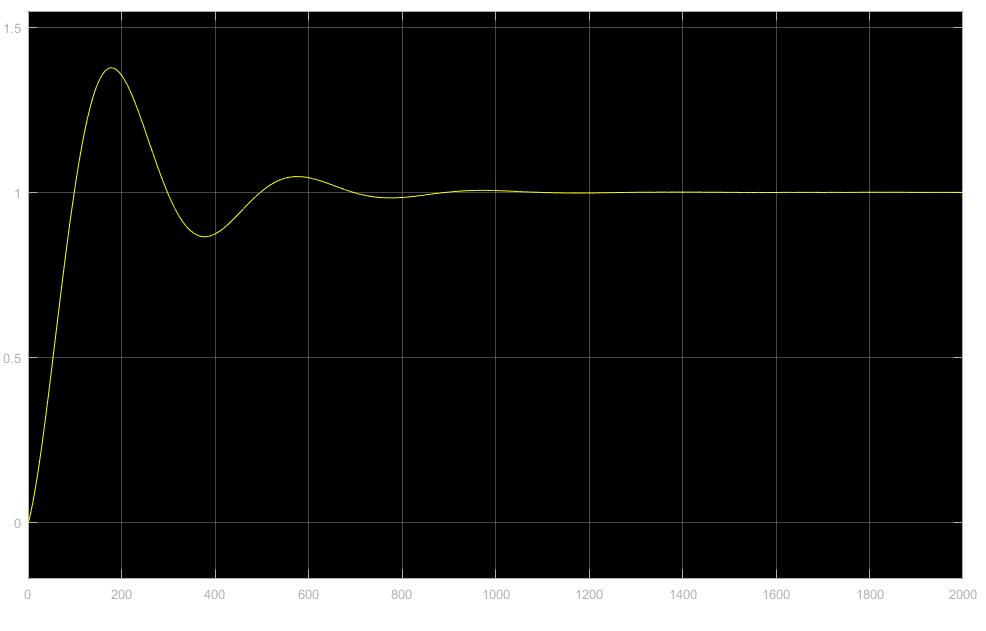4.2 当fundamental sample time 设置为0.1时，实验结果如下：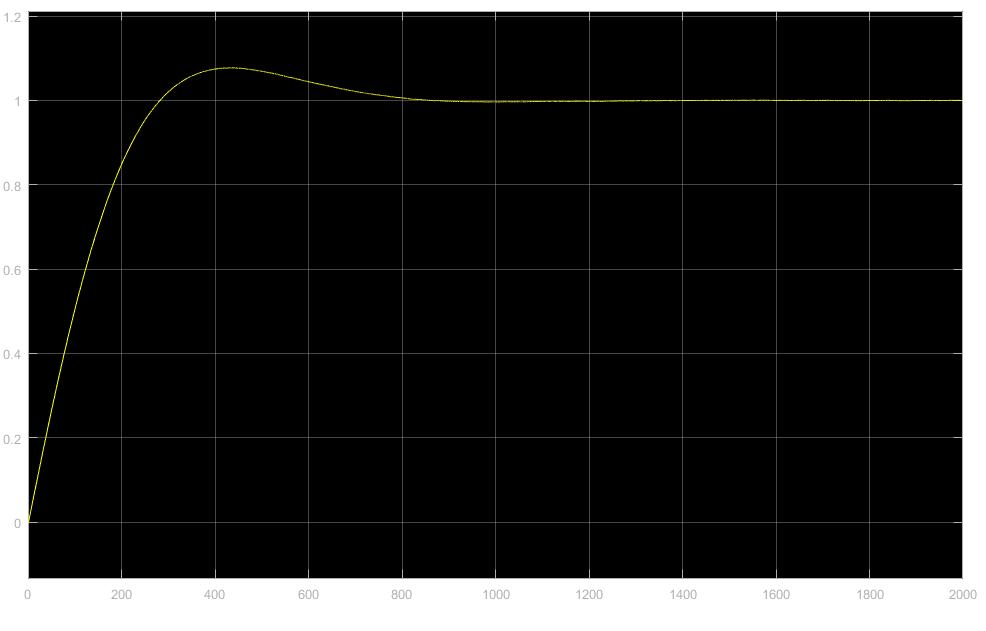最后，由于作者水平有限，感谢各位大家批评指正。另外，我将自己的代码链接代码附上。点击打开链接（https://download.csdn.net/download/zkzfengyi/10441272）
展开全文MATLAB

万次阅读 多人点赞 2019-05-30 10:58:30
最近在学习电机的智能控制，上周学习了基于单神经元的PID控制，这周研究基于BP神经网络PID控制。 神经网络具有任意非线性表达能力，可以通过对系统性能的学习来实现具有最佳组合的PID控制。利用BP神经网络可以...
    最近在学习电机的智能控制，上周学习了基于单神经元的PID控制，这周研究基于BP神经网络的PID控制。

神经网络具有任意非线性表达能力，可以通过对系统性能的学习来实现具有最佳组合的PID控制。利用BP神经网络可以建立参数Kp,Ki,Kd自整定的PID控制器。基于BP神经网络的PID控制系统结构框图如下图所示：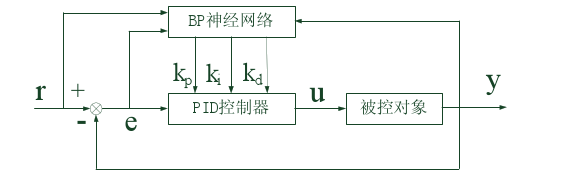控制器由两部分组成：经典增量式PID控制器；BP神经网络

经典增量式PID控制器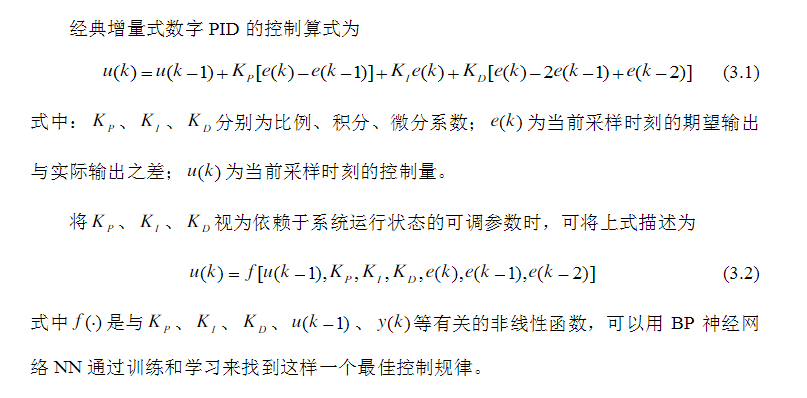BP神经网络控制算法

BP神经网络结构如下图所示：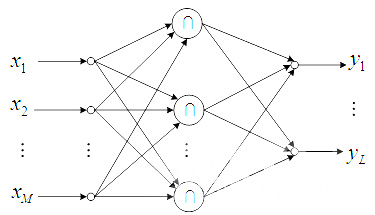它是一种有隐含层的3层前馈网络，包括输入层、隐含层和输出层。输出层的三个输出分别对应PID控制器的三个可调参数Kp、Ki和Kd。由于Kp、Ki和Kd不能为负，所以输出层神经元的变换函数取非负的Sigmoid函数，而隐含层神经元的变换函数可取正负对称的Sigmoid函数。

BP神经网络的输入（M为输入变量的个数）：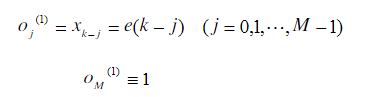隐含层的输入输出为：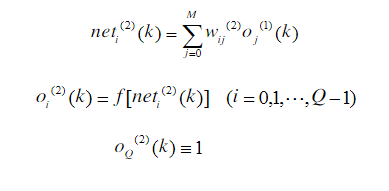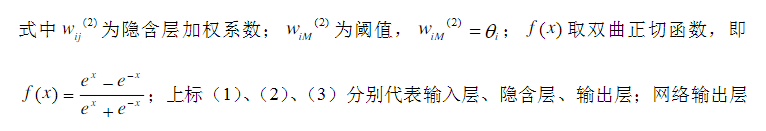输出层的输入输出：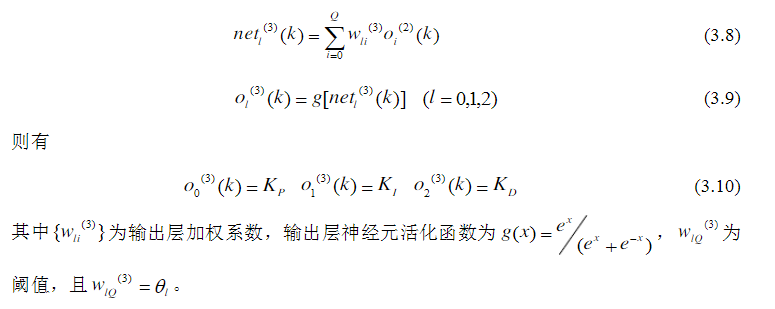采用以输出误差二次方为性能指标，其性能指标函数为：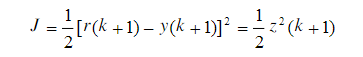按照梯度下降法修正网络的加权系数，并附加一使搜索快速收敛全局极小的惯性项，则有BP神经网络输出层的加权系数修正公式为：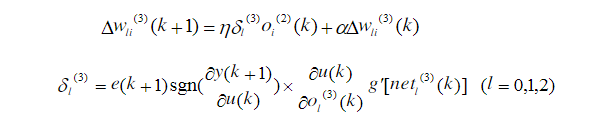同理，可得隐含层加权系数的计算公式为：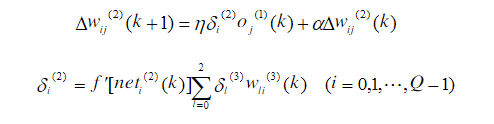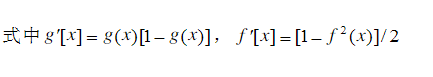由此，BP神经网络PID控制算法可总结为：

（1）确定BP神经网络结构，即确定输入层和隐含层的节点个数，选取各层加权系数的初值wij(0)、wli(0),选定学习速率和惯性系数，此时k=1

（2）采样给定和反馈信号，即r(k)和y(k),计算误差e(k)=r(k)-y(k)

（3）确定输入量

（4）根据上述公式，计算各层神经元的输入、输出，神经网络输出层即为PID控制器的三个可调参数Kp、Ki和Kd

（5）由增量式PID控制公式，计算PID控制器的控制输出u(k)

（6）进行神经网络学习，实时自动调整输出层和隐含层的加权系数wli(k)和wij(k)，实现PID控制参数的自适应调整

（7）置k=k+1，返回步骤（2）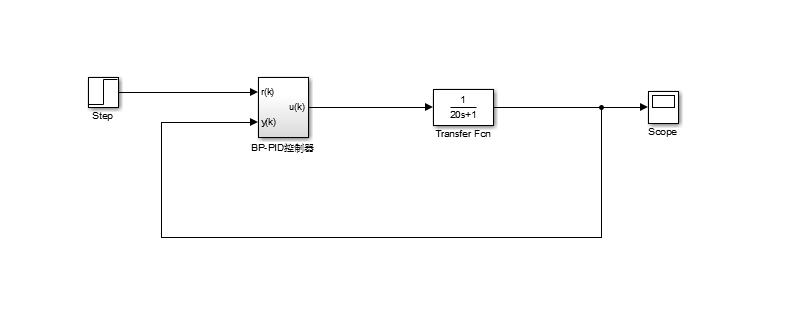输入为阶跃信号，其参数为默认值，一个简单的闭环控制系统。BP神经网络PID控制器的内部结构如下图所示：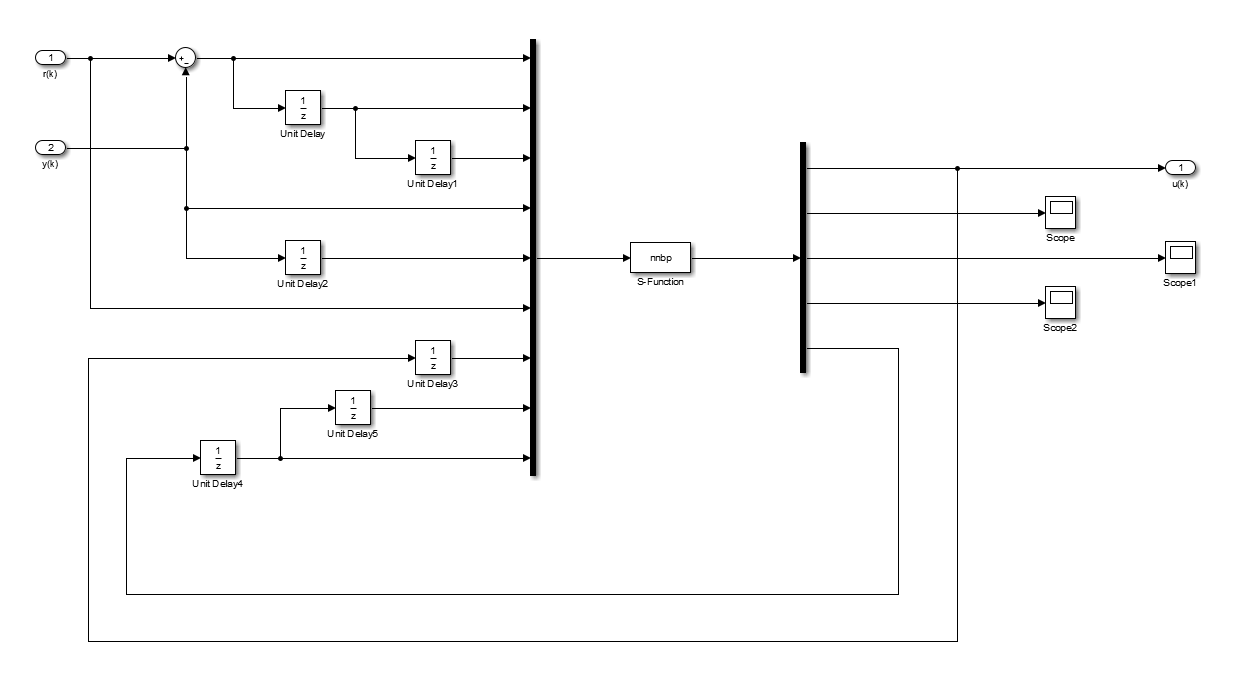S-function的输入为:u=[e(k);e(k-1);e(k-2);y(k);y(k-1);r(k);u(k-1);隐含层+输出层权值系数(k-2);隐含层+输出层权值系数(k-1)]=

[u(1);u(2);u(3);u(4);u(5);u(6);u(7);...u(隐含层权值个数+输出层权值个数）]，把所有的权值系数从输出再返回到输入是为了更新权值矩阵，从而自适应的调整PID三个参数。关于S-function的使用方法，请参考我写的另一篇博客：           https://blog.csdn.net/weixin_42650162/article/details/90488610

S-function函数

下面是S-function函数编写的控制算法：

为了更好的理解下面的程序代码，先要理解Matlab中的几个函数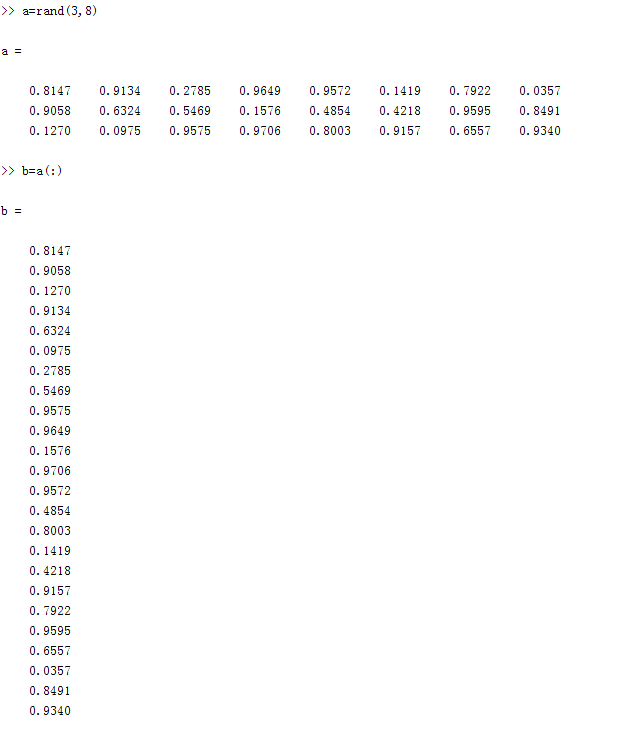通过(:)把一个矩阵变为一个列向量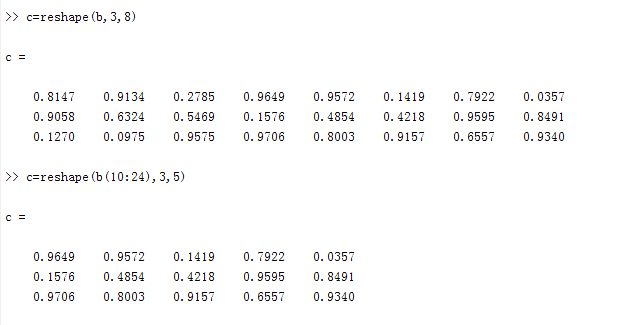通过reshape函数，从列向量里任意组成矩阵如c=reshape(b,3,8)，b中元素按顺序排成一个3*8的矩阵，也就是还原了矩阵a，

c=reshape(b(10:24),3,5)，b中第10个元素到第24个元素，按顺序排成一个3*5的矩阵。

在我编写的S-function函数中，就是通过reshape函数，把输入的隐含层+输出层的列权值系数还原成：隐含层权值系数矩阵+输出层权值系数矩阵，通过算法完成这两个权值系数矩阵的更新。

下面是M文件编写的S-function控制算法：

function [sys,x0,str,ts,simStateCompliance] = nnbp(t,x,u,flag,T,nh,xite,alfa)
switch flag,
case 0,
[sys,x0,str,ts,simStateCompliance]=mdlInitializeSizes(T,nh);
%初始化函数
case 3,
sys=mdlOutputs(t,x,u,nh,xite,alfa);
%输出函数
case {1,2,4,9},
sys=[];
otherwise
end
function [sys,x0,str,ts,simStateCompliance]=mdlInitializeSizes(T,nh)
%调用初始画函数，两个外部输入参数 参数T确定采样时间，参数nh确定隐含层层数
sizes = simsizes;
sizes.NumContStates  = 0;
sizes.NumDiscStates  = 0;
sizes.NumOutputs     = 4+6*nh;
%定义输出变量，包括控制变量u,三个PID参数：Kp,Ki,Kd,隐含层+输出层所有加权系数
sizes.NumInputs      = 7+12*nh;
%定义输入变量，包括前7个参数[e(k);e(k-1);e(k-2);y(k);y(k-1);r(k);u(k-1)]
%隐含层+输出层权值系数（k-2),隐含层+输出层权值系数（k-1）
sizes.DirFeedthrough = 1;
sizes.NumSampleTimes = 1;
sys = simsizes(sizes);
x0  = [];
str = [];
ts  = [T 0];
simStateCompliance = 'UnknownSimState';
function sys=mdlOutputs(t,x,u,nh,xite,alfa)
%调用输出函数
wi_2 = reshape(u(8:7+3*nh),nh,3);
%隐含层（k-2)权值系数矩阵，维数nh*3
wo_2 = reshape(u(8+3*nh:7+6*nh),3,nh);
%输出层（k-2）权值系数矩阵，维数3*nh
wi_1 = reshape(u(8+6*nh:7+9*nh),nh,3);
%隐含层（k-1)权值系数矩阵，维数nh*3
wo_1 = reshape(u(8+9*nh:7+12*nh),3,nh);
%输出层（k-1）权值系数矩阵，维数3*nh
xi = [u(6),u(4),u(1)];
%神经网络的输入xi=[u(6),u(4),u(1)]=[r(k),y(k),e(k)]
xx = [u(1)-u(2);u(1);u(1)+u(3)-2*u(2)];
%xx=[u(1)-u(2);u(1);u(1)+u(3)-2*u(2)]=[e(k)-e(k-1);e(k);e(k)+e(k-2)-2*e(k-1)]
I = xi*wi_1';
%计算隐含层的输入，I=神经网络的输入*隐含层权值系数矩阵的转置wi_1'，结果为：
%I=[net0(k),net1(k)...netnh(k)]为1*nh矩阵
Oh = exp(I)./(exp(I)+exp(-I));
%激活函数，可更改
%计算隐含层的输出，(exp(I)-exp(-I))./(exp(I)+exp(-I))为隐含层的激活函数Sigmoid
%Oh=[o0(k),o1(k)...onh(k)],为1*nh的矩阵
O = wo_1*Oh';
%计算输出层的输入，维数3*1
K = 2./(exp(O)+exp(-O)).^2;
%激活函数，可更改
%计算输出层的输出K=[Kp,Ki,Kd]，维数为1*3
%exp(Oh)./(exp(Oh)+exp(-Oh))为输出层的激活函数Sigmoid
uu = u(7)+K'*xx;
%根据增量式PID控制算法计算控制变量u(k)
dyu = sign((u(4)-u(5))/(uu-u(7)+0.0000001));
%计算输出层加权系数修正公式的sgn
%sign((y(k)-y(k-1))/(u(k)-u(k-1)+0.0000001)近似代表偏导
dK = 2./(exp(K)+exp(-K)).^2;
%激活函数，可更改
delta3 = u(1)*dyu*xx.*dK;
wo = wo_1+xite*delta3*Oh+alfa*(wo_1-wo_2);
%输出层加权系数矩阵的修正
dOh = 2./(exp(Oh)+exp(-Oh)).^2;
%激活函数，可更改
wi = wi_1+xite*(dOh.*(delta3'*wo))'*xi+alfa*(wi_1-wi_2);
%隐含层加权系数修正
sys = [uu;K(:);wi(:);wo(:)];
%输出层输出sys=[uu;K(:);wi(:);wo(:)]=
%[uu;Kp;Ki;Kd;隐含层+输出层所有权值系数]
%K(:),wi(:),wo(:),把这三个矩阵按顺序排为列向量

本函数有四个外部输入变量：T，nh，xite,alfa T输入采样时间，nh确定隐含层层数，xite和alfa权值系数修正公式里的学习速率和惯性系数。

为了更好地分配S-function的输出，需要对Demux进行如下设置：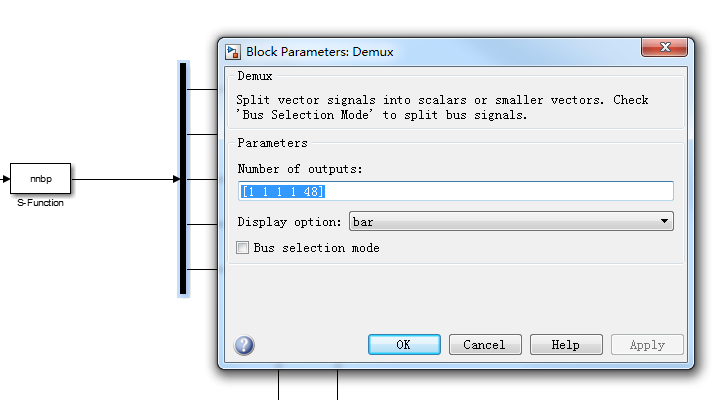确保前三个输出变量为：控制变量u,Kp,Ki,Kd,剩下的变量为隐含层权值系数矩阵+输出层权值系数矩阵总数之和。

然后对此S-function函数进行封装：

具体过程可以参考我的另一篇博客：

https://blog.csdn.net/weixin_42650162/article/details/90488610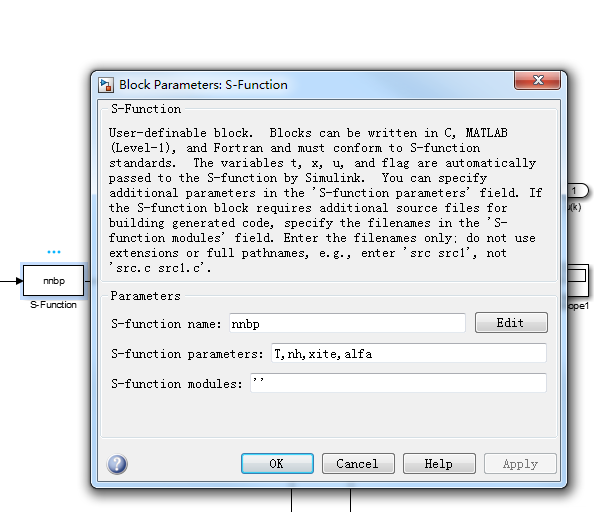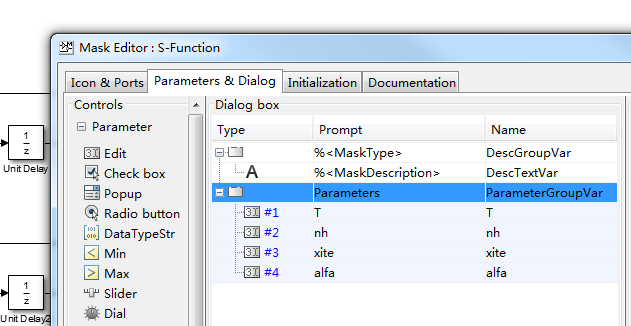完成后点击S-function函数，会弹出外部参数设置框，设置参数如下：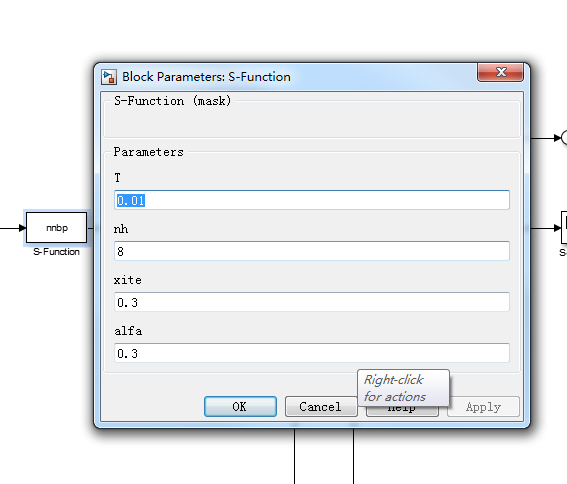注：T采样时间要和设置的控制算法的采样时间一样，不然会报错，如下：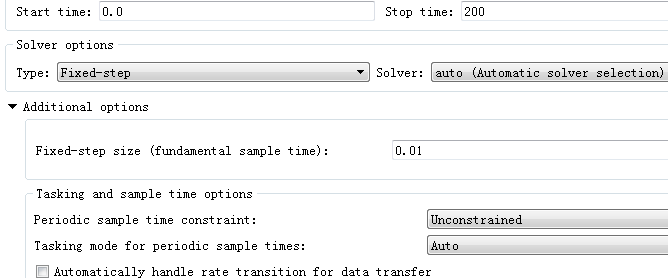下面是仿真结果：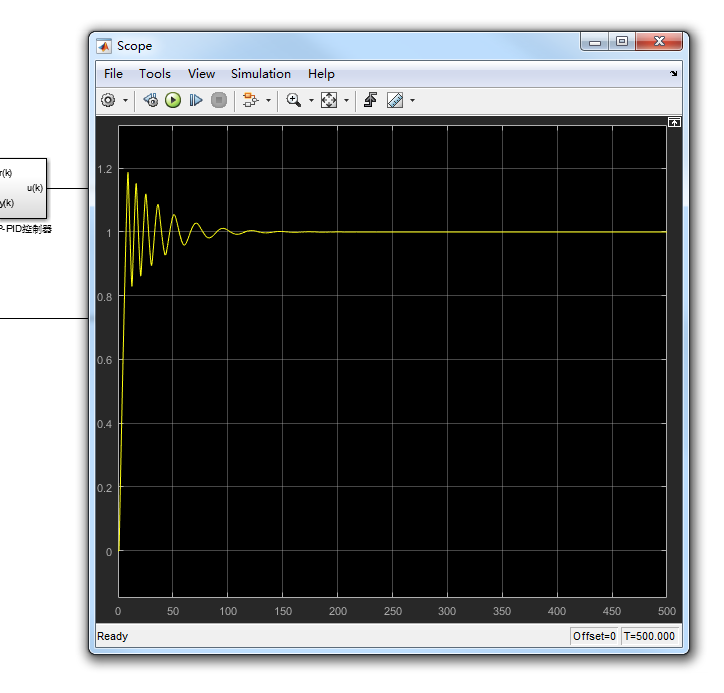Kp、Ki、Kd的自适应变化曲线：

Kp：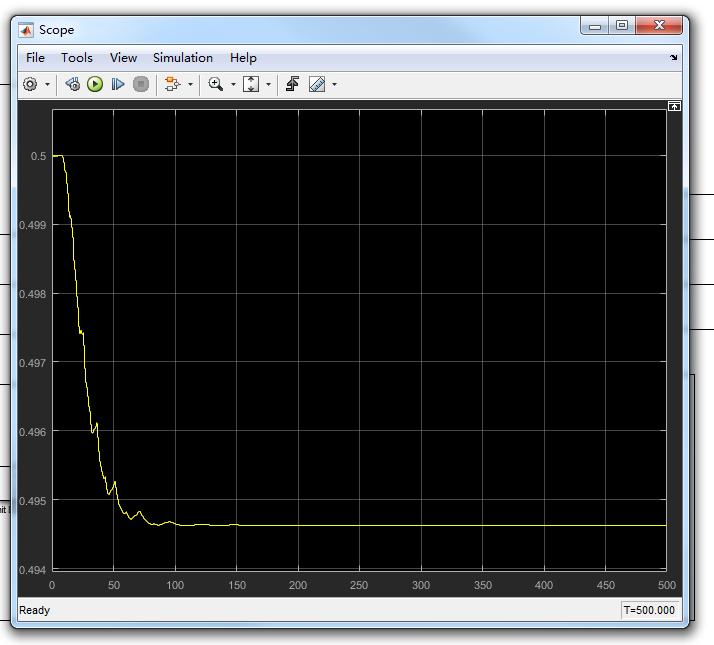Ki：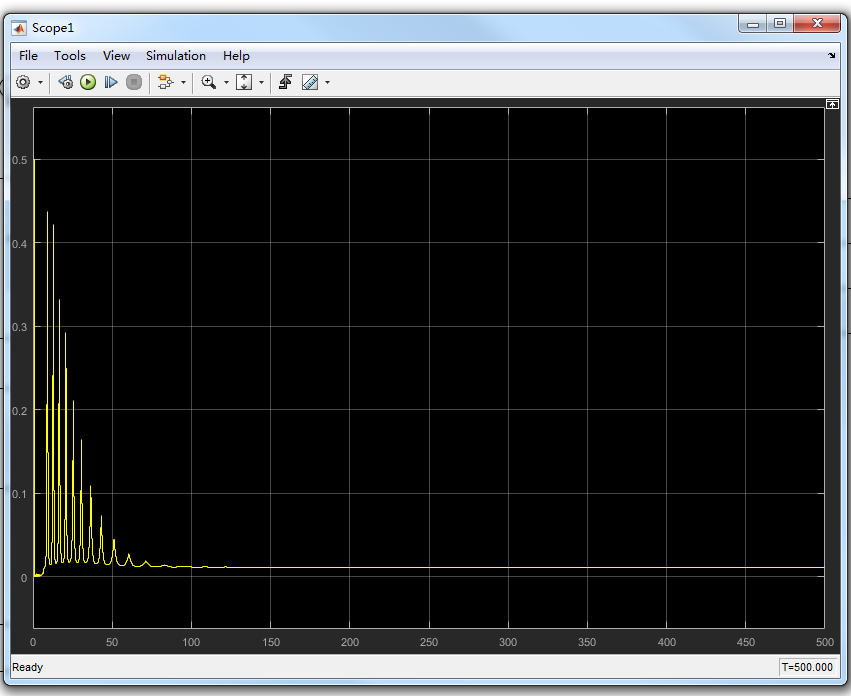Kd: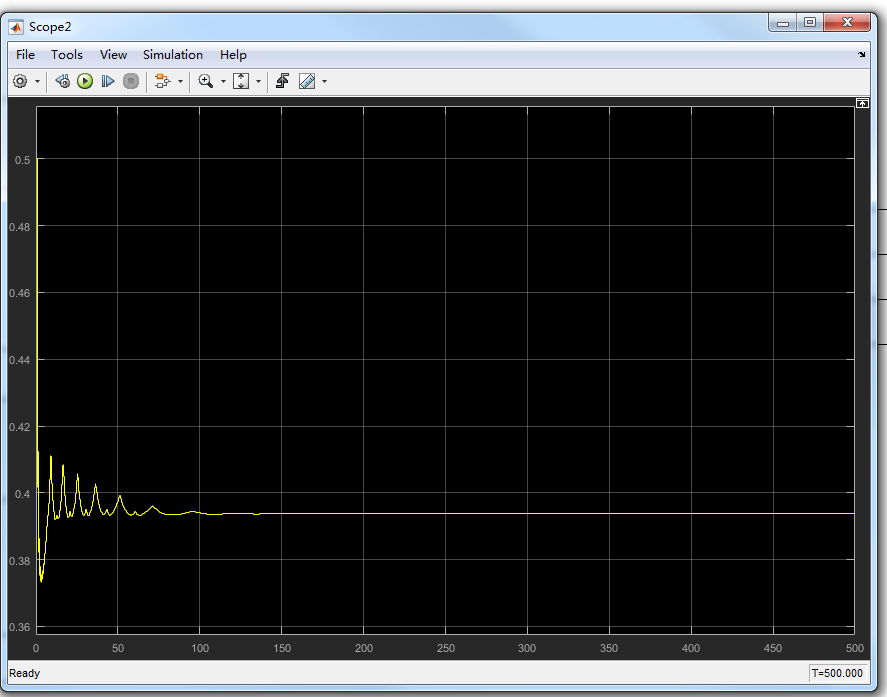有关仿真的所有资源已上传，如有需要可自行下载：


展开全文Simulink仿真 智能控制 控制系统
• 参考： https://blog.csdn.net/qq_40794710/article/details/98786347
参考：
https://blog.csdn.net/qq_40794710/article/details/98786347


展开全文• %% 基于bp神经网络pid控制程序 %% BP based PID Control %% 清除环境变量和命令行的内容 clear all; close all; clc; %% 参数的设置，正弦信号与阶跃信号对应的权值的初始设定 xite=0.25; % 学习速率 a....simulink
• BP神经网络整定的PID算法_matlab源程序，神经网络的PID算法，MATLAB源程序代码
• 参考： https://blog.csdn.net/weixin_42650162/article/details/90678503simulink仿真
• BP神经网络PID控制算法在三容水箱系统中的研究与应用
• 一个基于BP神经网络PID控制，《先进PID控制及其MATLAB仿真》一个例子
• BP神经网络完成PID参数自适应，这是其中由M文件编写的S函数
• 该论文给出了控制器的算法的实现，是用matlab中的S函数写的，而且还给出了仿真图，以及封装图等等，对大家肯定有很大的帮助
• BP PID控制器，加入了一个传递函数作为案例，能够实现优化PID算法，
• 5篇关于bp神经网络pid的学术论文。对于刚做刚接触神经网络控制的童鞋是有很大帮助的matlab